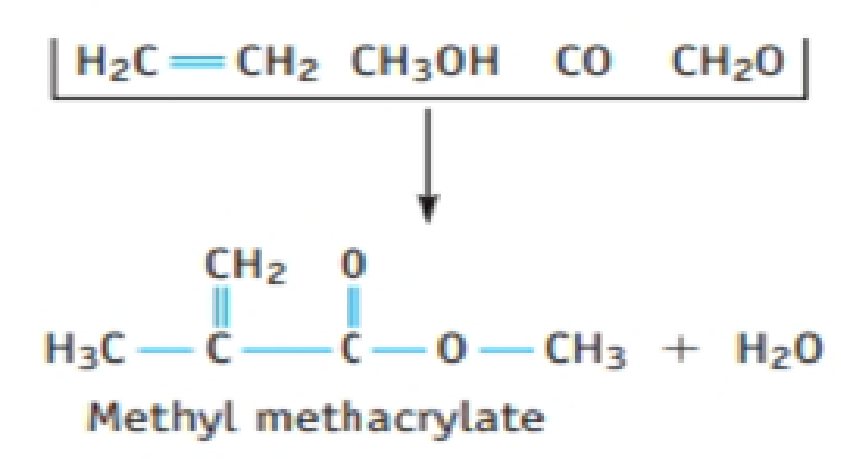Chapter 4.9, Problem 1.1ACP

Chapter
Section
Textbook Problem

Methyl methacrylate is used to prepare plastics you may know as Lucite or some other tradename. The substance was introduced in 1933, but chemists have long sought better (and less costly) ways to make the compound. In a newly developed process, ethylene (C2H4), methanol (CH3OH), CO, and formaldehyde (CH2O) combine in two steps to give methyl methacrylate and water. What is the atom economy of this new process?Interpretation Introduction

Interpretation:

The atom economy of the given reaction has to be determined.

Concept introduction:

• Atom economy is one of the key parameter used to evaluate the efficiency of a reaction.

%atomeconomy=molarmassofatomutilizedmolarmassofreactants×100%

• The molar mass of an element or compound is the mass in grams of 1 mole of that substance, and it is expressed in the unit of grams per mol (g/mol).
Explanation

Atom economy is one of the key parameter used to evaluate the efficiency of a reaction. Atom economy percentage of a reaction can be calculated by using the following equation.

%atomeconomy=molarmassofatomutilizedmolarmassofreactants×100%

In the given reaction a new product methyl methacrylate is prepared from ethylene

(H2C=CH2), methanol (CH3OH), carbon monoxide (CO) and formaldehyde (CH2O)

The reaction can be represented as follows,

H2C=CH2CH3OHCOCH2O

Still sussing out bartleby?

Check out a sample textbook solution.

See a sample solution

The Solution to Your Study Problems

Bartleby provides explanations to thousands of textbook problems written by our experts, many with advanced degrees!

Get Started